# Spectral Line Workshop

## NGC660 Hydrogen Absorption

In the second of the Unit 4 DARA tutorials, we shall be reducing a spectral line dataset taken with the European VLBI Network. The aim of this tutorial is to introduce the various extra calibration and imaging steps associated with spectral line data. In addition, we will focus on extracting some science from the results (something which was missing from tutorial one). This workshop was originally curated by Anita Richards and has been subsequently updated my Jack Radcliffe. This tutorial should only be conducted using CASA v6.3 or above. If you want to use the CASA v5 version of this tutorial instead then follow this link.
Please use the contact form at the bottom of the page should you find any issues or have any general questions about this workshop. In you are happy, then please follow the instructions below!

Before we start we need to ensure that these data and scripts are all there. Note that this tutorial should only take up around 7-10 GB on your hard drive. These files should already be on your filesystem (ask the trainers!) unless you are doing this remotely then you should use the following buttons to download these data. If your internet is slow, download the small tar ball i.e. NGC660 data (624MB). The files included are those with the * and the other data files can be generated from these files. If your internet is fast, download the full tar-ball i.e. NGC660 data all (1.7GB):

Unpack the tar file and you should find the following files in your directory:

• *NGC660.FITS* - UVfits data set with instrumental and calibration source corrections applied - needed to run from start of this script.
• NGC660shift.ms.tar.gz - data set for starting at step 8 (you make this yourself if working through steps 1-7).
• NGC660_line.clean.image.tar.gz and NGC660_contap1.clean.image.tar.gz - images for analysis (you will make these yourself, but just in case...).
• *NGC660.py* - script covering the steps in this web page.
• *NGC660.flagcmd.txt* - flags for step 2

In this tutorial, we will be reducing some spectral line data of the starburst galaxy NGC660. You will notice that many of the steps are similar like before, however there are some extra complications. Before we start, please follow the instructions to download the relevant data sets and scripts and then we can begin. The guide starts with a data set which has already been flagged and calibrated using the instrumental corrections and calibration derived from astrophysical sources including phase referencing. This was done in AIPS and the split-out, calibrated data NGC660.FITS are in UVFITS format.

## 1. Background on NGC660

NGC660 is an odd starburst galaxy with a polar ring located approximately 13.5 Mpc away. This weird configuration is likely due to a major merger event of two galaxies around one billion years ago or the capture of matter from a nearby galaxy which then formed the ring structure.

.The central area contains a region of intense star-formation. Radio observations originally suggested that the centre of the galaxy could be a super-cluster of stars, however between 2008 and 2012 this all changed. Observations detected an outburst which was around 10 times as bright as a supernovae explosion. A new continuum radio source appeared along with absorption lines which suggested that this new source originated from the accretion of matter on the central black hole. These observations using the European VLBI Network were designed to determine and confirm the Active Galactic Nuclei origin of this source and investigate any inflows/ outflows of neutral gas via HI absorption lines. It is this data that we will be reducing in this tutorial. To read more about the results of these observations see Argo et al. 2015.

## 2. Improving the calibration of NGC660

In order to use this Guide, you can either run the script (NGC660.py) step by step, or cut and paste each task from this web page, or enter parameters line by line (in which case omit the comma at the end of each line). If you are working from this web page you need to enter some values yourself, but these are in the script. Please make sure that you try to work out what each step does otherwise you will learn nothing!.

The Guide starts with a data set which has already been flagged and calibrated using the instrumental corrections and calibration derived from astrophysical sources including phase referencing.

### A. Convert data to MS and get information (step 1)

These data are from AIPS, which exports visibilities in UVFITS format. We therefore need to convert these from UVFITS to a measurement set using the CASA task importuvfits

os.system('rm -rf NGC660.ms*')
importuvfits(fitsfile='NGC660.FITS',
vis='NGC660.ms')

As normal, one of the tenets of good data reduction is understanding the data. Let's use listobs to see what is in the data.

os.system('rm -rf NGC660.ms.listobs')
listobs(vis='NGC660.ms',
listfile='NGC660.ms.listobs')

Take a look at the listobs output to see what is in these data. You should see that there is just one source, one spectral window (spw) with 1024 channels. In total, there are 7 antennas.

### B. Plot visibility spectrum, identify continuum, flag bad data (step 2)

To start, we need to identify where the continuum emission and spectral line features are within the band. This is to ensure that we can successfully subtract the continuum emission from the spectral line absorption/emission and also ensure that we do not mistake the spectral line with RFI and flag it during the process. In most projects with spectral line data, the proposal for the project will include the spectral lines we are searching for in these data, so you'd be able to glean this information from there. However, in the absence of this information, there are two ways we can find the location of the spectral lines.

If the spectral line is strong enough (as is the case in this data), we can plot the data (and average in time) and we should be able to see the lines. However, for weak lines, we must use the distance of the source to estimate the location of the lines in frequency space. We shall do this for this data set for completeness. NGC660 is around 13.5 Mpc away which corresponds to a redshift of 0.003. We are searching for HI absorption, whose rest frame frequency is 1420.405751 MHz, therefore our observed line frequency should be near to $1.420405751/1+z = 1.4160961\,\mathrm{GHz}$. Note that the rest frame frequencies of spectral lines can be found using the Splatalogue database or the slsearch CASA task.

Luckily, in our dataset, the absorption feature is bright so we can estimate the location of the line directly from the data. We will use plotms to visualise this. As before, EF (Effelsburg) is the most sensitive antenna and is used as the reference antenna. We will plot amplitude against channel. The end channels and some around channel 775 have already been been flagged. The absorption can be seen clearly and has two components.

## In CASA
plotms(vis='NGC660.ms',
xaxis='channel',
yaxis='amp',
avgtime='36000',
antenna='EF&*',
iteraxis='baseline',
avgscan=True)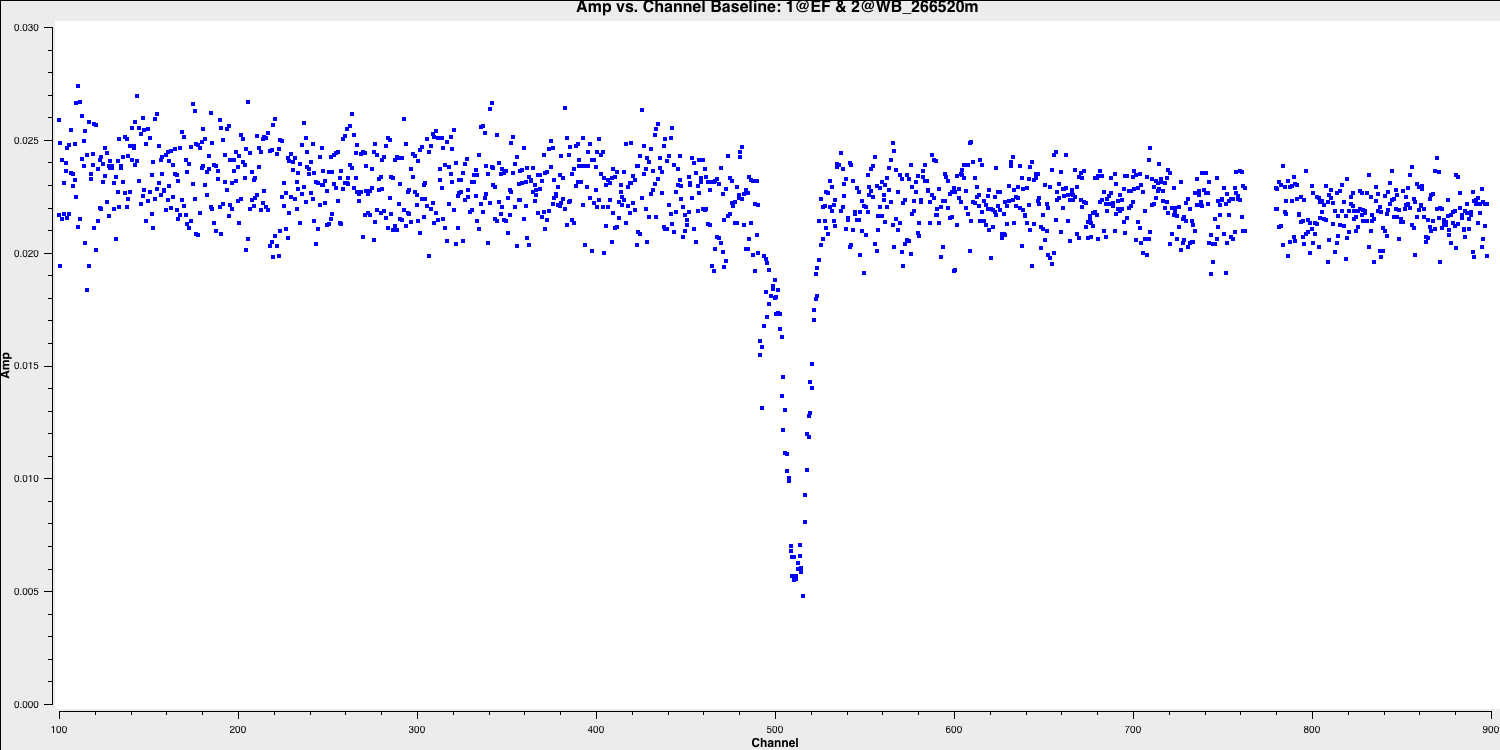Select the continuum channel ranges. As you can see, the spectral line deviates from the expected frequency of $1.416\,\mathrm{GHz}$ we calculated earlier and has two components. This is because the absorption regions have their own intrinsic velocity towards and away us along the line of sight. This widens the region we want to classify as spectral line within the spw. We therefore want to exclude the 100 channels around the line and define this as the continuum emission region using the variable contchans (fill in xxx and yyy). Enter this into CASA:

contchans='0:100~xxx;yyy~900'

In order to ensure that we get the best images of our line we should check for any further bad data. Remember that flagging is an iterative process and some low-level bad data will only appear after you partially calibrate the data. In these data, there was intial flagging done before phase referencing but the calibration of the target has made some more bad data appear.

Let's plot the data to identify the bad data, and we shall use Mark and Locate to see on which baselines/antennas this is on. Note that we do not want to flag the high data as these are mostly just mis-scaled and will be calibrated later.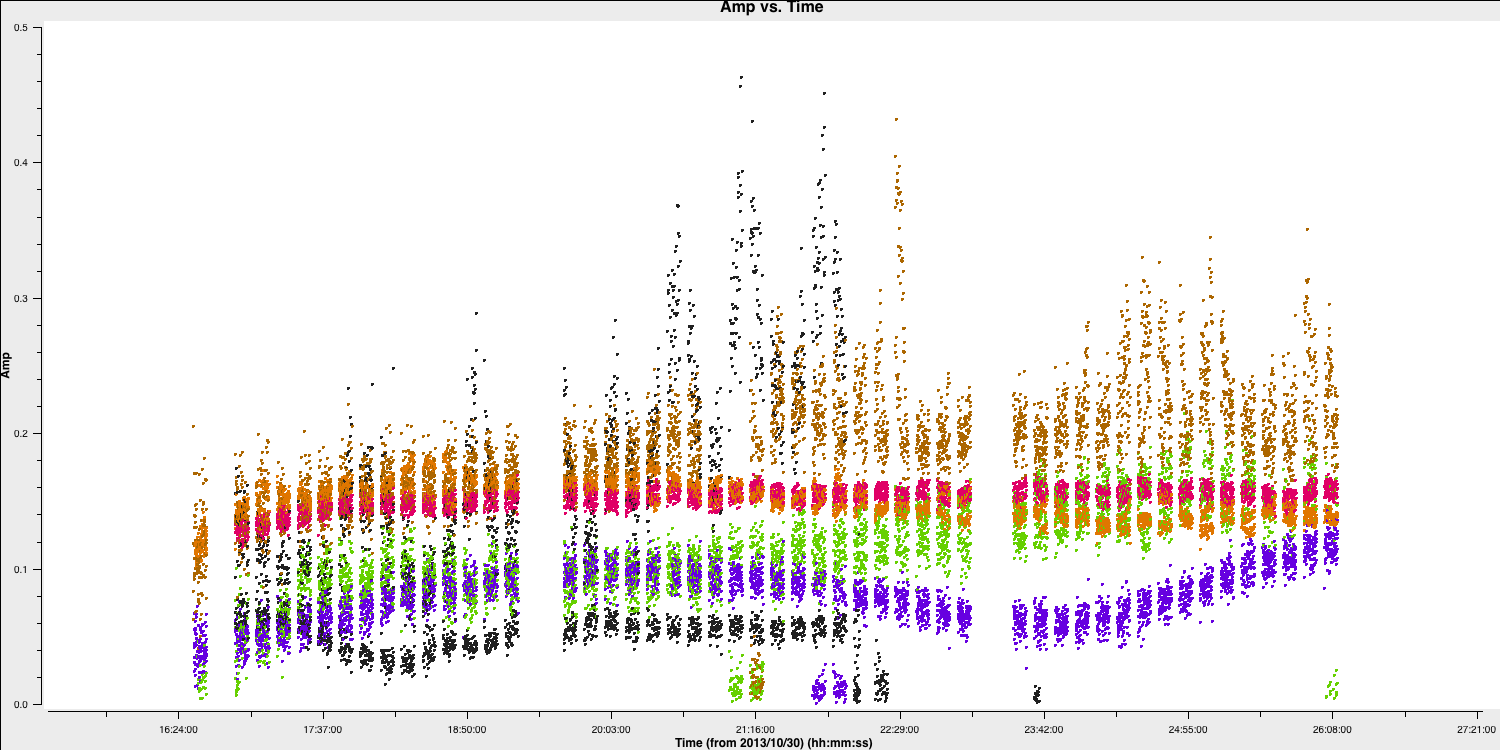The bad data here are the scans where the visibilities drop to zero amplitude. These are on a variety of scans and you should find that they are mainly on the Onsala (ON) and Medicina (MC) telescopes and is mainly confined to single polarisations. Normally when you flag data you would want to make a flag file to record this which enables you to exactly replicate the flagging if you need to re-calibrate these data.

To save time, we have provided the flag file already made. Use your favourite text editor to open NGC660.flagcmd.txt. This file contains the commands to flag these data and compare this to the information you received by using the Mark and Locate function of plotms task.

If you are happy that these conform then we can use the flagfile to flag these bad data. We shall use flagmanager to create a backup of the current flags (in case we need to go back!) and then use flagdata to apply the flagfile:

flagmanager(vis='NGC660.ms',
mode='save',
versionname='prelist')

flagdata(vis='NGC660.ms',
mode='list',
inpfile='NGC660.flagcmd.txt')

With this applied, we should reload plotms to ensure that all of the bad data is gone (use the tget command!)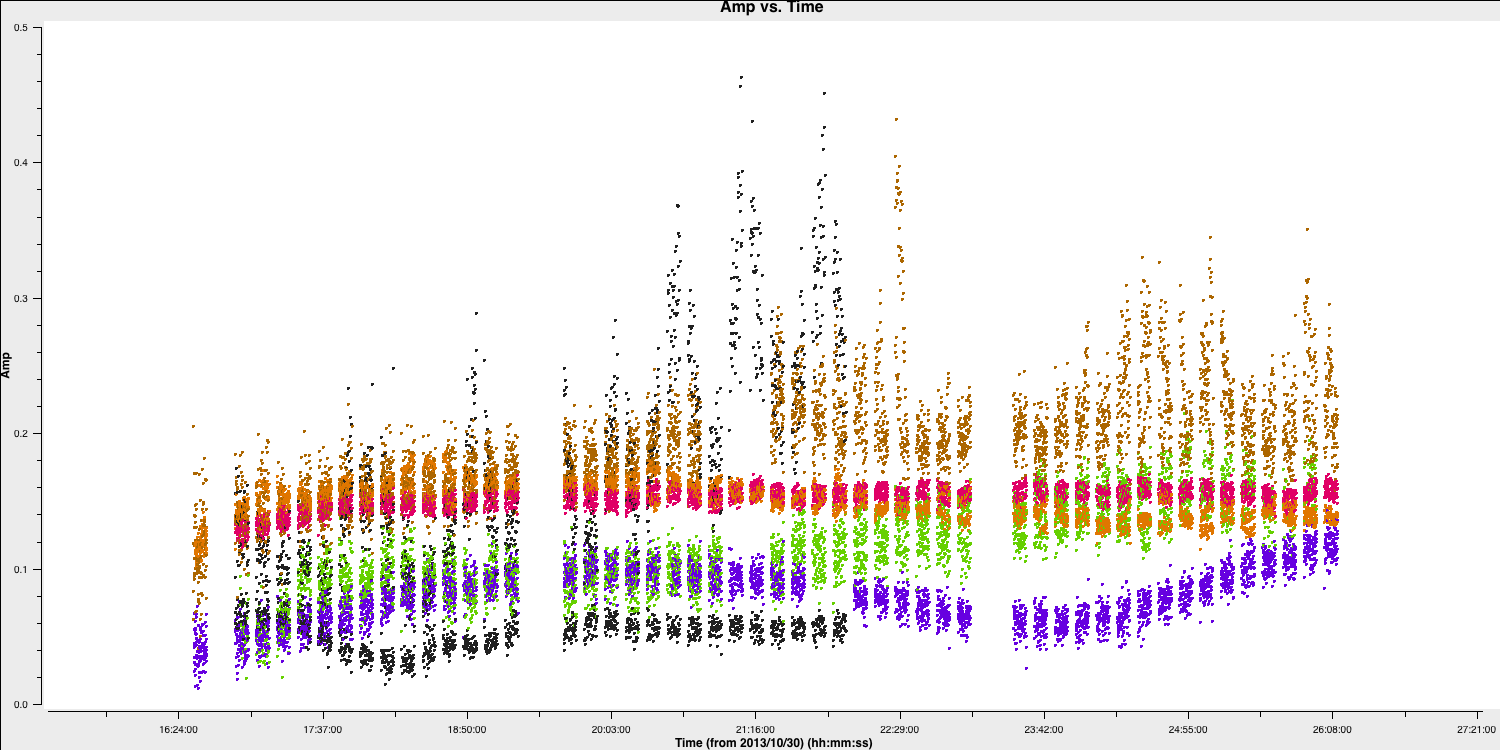Hooray all of the bad data is gone!

### C. Plot amplitude versus uv (step 3)

As we have excised the bad data and the phase referencing has already been conducted, we should prepare to conduct our first imaging run so that we can perform self-calibration on these data. The first thing we need to do is determine the imaging parameters. The most important thing is to determine the cell parameter which can be done from the amp vs. uv plot:

plotms(vis='NGC660.ms',
xaxis='uvwave',
yaxis='amp',
avgchannel='801',
avgtime='30',
coloraxis='antenna1',
overwrite=True)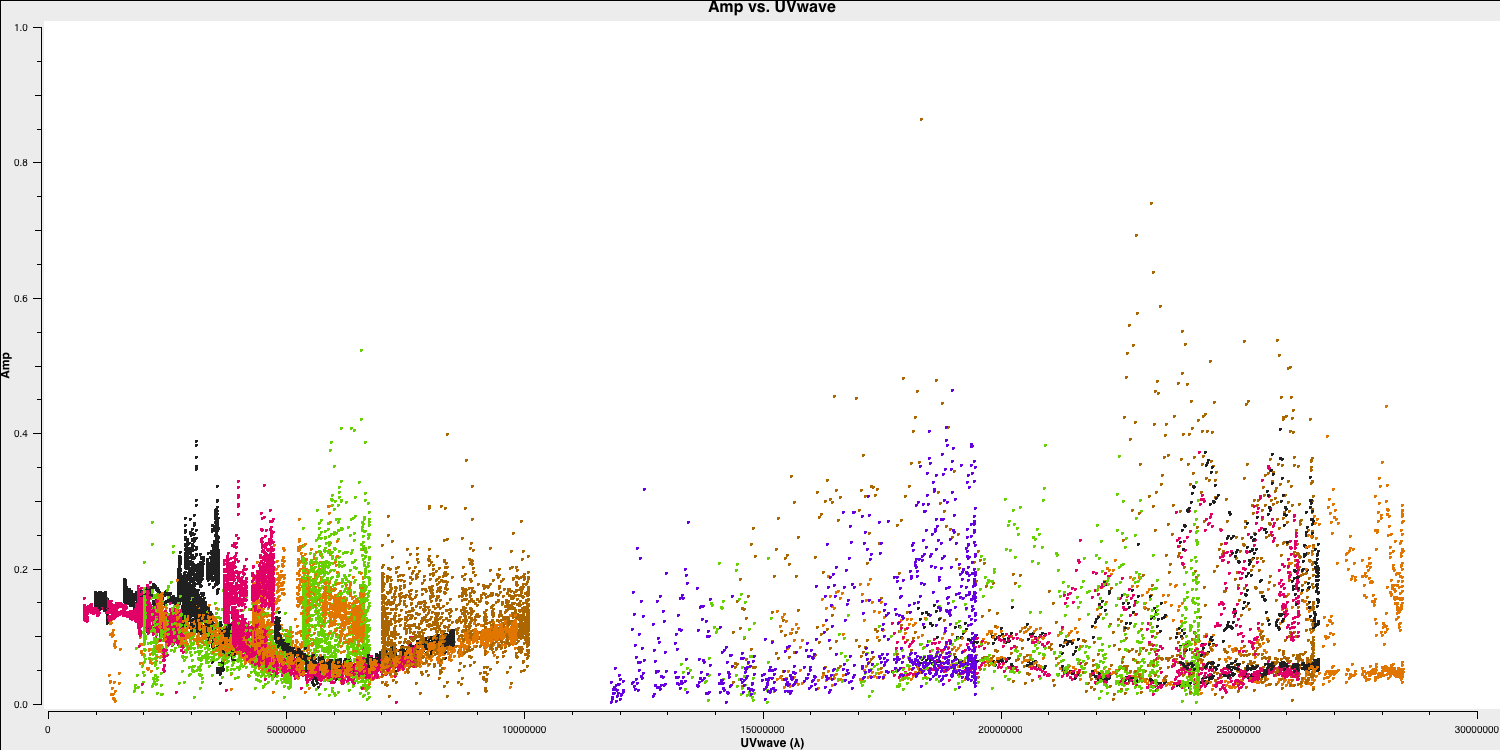Remember from part 2 of the EVN continuum tutorial that we used this plot to estimate the effective resolution of our interferometer given that the resolution of an interferometer is given by $\lambda/\mathrm{B}$ where $\mathrm{B}$ is the max. baseline length. Based upon this, we derived the following equation to obtain the cell size (including the Nyquist sampling parameter $N_\mathrm{s}$ so that we can fit to the PSF). $$\mathrm{cell} \approx \frac{180}{\pi N_{\mathrm{s}}} \times \frac{1}{D_{\max }[\lambda]}[\mathrm{deg}]$$ When you have worked this out, set the cellsize variable in CASA or in the script (it should be between 1.5 and 2.5 milliarcseconds depending on your choice of $N_\mathrm{s}$):

cellsize='xxxmas'

### D. Make the first continuum image (step 4)

As with many projects, we don't know exactly where the target source is. In this case, the target position was only known to around an astrometric accuracy of around one arcsecond. We therefore want to make a larger image to obtain the target location. We shall use an image size of 1280 (an optimal number for the underlying algorithms i.e. $2^n \times 10$). In addition, we want to avoid the spectral line at the moment so we use the contchans parameter to define the spectral windows.

os.system('rm -rf NGC660_cont0.clean*')
tclean(vis='NGC660.ms',
imagename='NGC660_cont0.clean',
field='NGC660',
spw=contchans,
deconvolver='clark',
imsize=[1280,1280],
cell=cellsize,
weighting='natural',
niter=100,
interactive=True,
cycleniter=25)

You should notice that the source is offset from the pointing centre and we will adjust this soon. Clean the dirty image to generate a clean image which should look something like the following.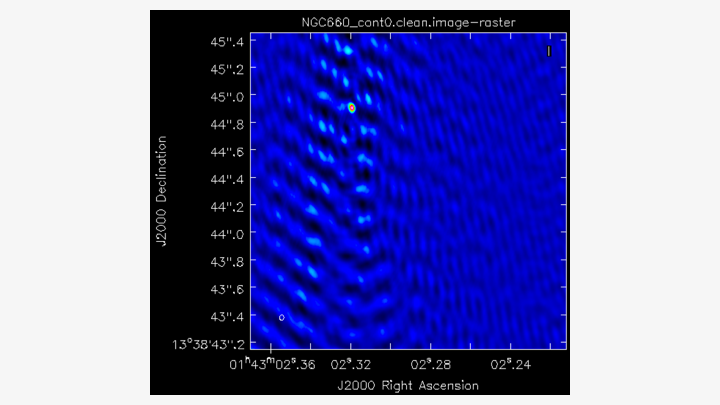### E. Find the source position (step 5)

Open the image in the viewer to obtain the same image as above. We want to adjust our visibilities so that the peak of the emission is at the centre of the image. This makes it easier to remove the continuum emission from the spectral line. CASA performs a phase shift to adjust the phase centre of the observation. Firstly, however, we need to work out the location of the peak brightness.

We can do this two ways, we can draw an region round the source and fit a 2-D Gaussian component interactively using the Region Fit tab as shown below: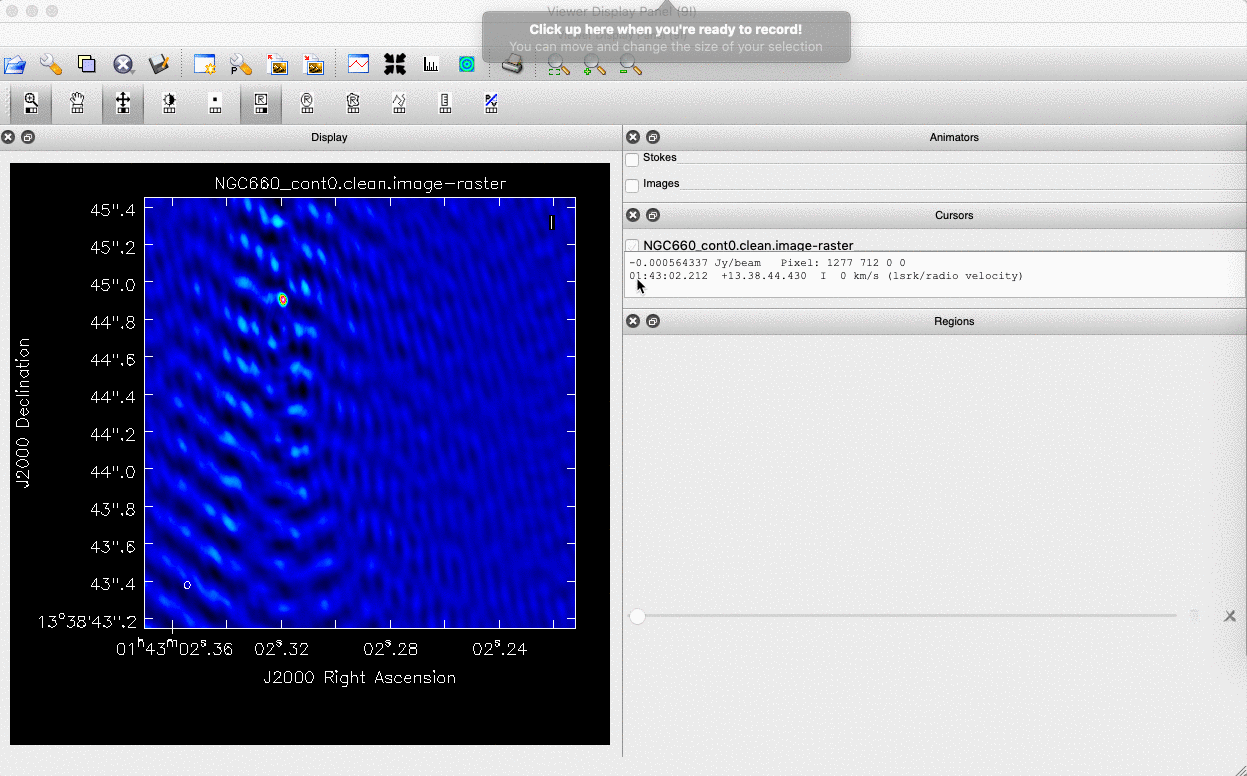One important aspect of this method is that we obtain our position from the logger and not the viewer panel. This is because the accuracy of the returned position is not sufficient in the viewer panel for VLBI purposes.

The second way is to use the task imfit to perform the same fitting. The advantage of this method is that we can put this in a script to do it automatically should this step need to be conducted again. To use the imfit task we have to define a box around the source where the source fitting shall be conducted. This is inputted into imfit as box='blc x, blc y, trc x, trc y' where trc is the top right corner and blc is the bottom left corner.

Use the viewer to determine these positions and set the variable boxsize as this. Next, lets run imfit with the following code:

imfit(imagename='NGC660_cont0.clean.image',
box=boxsize,
logfile='NGC660_contpeakpos.txt')

With either of these methods you should recover a peak brightness position of the source to be near to $\mathrm{RA} \approx 01^{h}43^{m}02.319403^{s}$ and $\mathrm{Dec} \approx +13^{d}38^{m}44.905554^{s}$. In the next step, we shall shift our data set to this position.

### E. Shift the uv phase to centre and make an image (step 6-7)

The task phaseshift is used to calculate and apply the phase shifts needed to place the source at the centre of the field, which makes continuum subtraction more accurate as well as making imaging more convenient. We need to apply our position derived earlier and set the phasecenter parameter.

phaseshift(vis='NGC660.ms',
outputvis='NGC660shift.ms',
phasecenter='J2000 HHhMMmSS.SSSSSS +DDdMMmSS.SSSSSS')

This task creates a new measurement set called NGC660shift.ms which has the source at the centre of the field. We shall use this measurement set from now on and we should get round to imaging it:

tclean(vis='NGC660shift.ms',
imagename='NGC660_cont1.clean',
field='NGC660',
spw=contchans,
deconvolver='clark',
imsize=[320,320],
cell=cellsize,
weighting='natural',
niter=50,
interactive=True,
cycleniter=25)

Clean this image and you should see that the source is now centred. Note that we can use a smaller image size as we have found the source and can see that it is compact. This reduces the computing time when imaging. Finally, we need to generate the MODEL data column so we use the ft task:

ft(vis='NGC660shift.ms',
field='NGC660',
model='NGC660_cont1.clean.model',
usescratch=True)

We are now ready to perform self-calibration, but firstly we want to track the improvement in image fidelity that is obtained with each calibration step. The easiest way is to track the signal to noise ratio (S/N) which improves with better calibration. We can do this automatically using the imstat task. We need to define two boxes, one which is off source to measure the rms noise and one on source to measure the peak brightness. Remember you can use imview to do this. The ratio of these gives us the S/N ratio.

rms=imstat(imagename='NGC660_cont1.clean.image',
box='offblcx,offblcy,offtrcx,offtrcy')['rms'] # Set blc and trc of a largeist box well clear of the source
peak=imstat(imagename='NGC660_cont1.clean.image',
box='blcx,blcy,trcx,trcy')['max'] # Set blc and trc to enclose the source
print('Peak %6.3f, rms %6.3f, S/N %6.0f' % (peak, rms, peak/rms))

Note that the notation for box is the same as what we used for imfit

You should obtain a peak brightness, $S_\mathrm{p} \approx 0.116\,\mathrm{Jy\,beam^{-1}}$, rms noise, $\sigma \approx 0.009\,\mathrm{Jy\,beam^{-1}}$ and so a $\mathrm{S/N}\approx 12$.

### F. Self-calibrate (step 8)

The visibility amplitudes show that there is good signal to noise on all baselines to EF. The data are mostly well calibrated but there are the discrepant amplitudes noted above, so solve for amplitude and phase. Note that the parallactic angle correction was applied prior to creating the data set we loaded. Looking at the zoomed plot of amplitude against time at the end of Step 2, the systematic trends in the amplitude errors seem to be greater than the noise scatter on timescales between 20-60 sec, so pick a solution interval in this range. We need to set solnorm=True because we have already done the fluxscaling in the pre-processing steps before this workshop.

gaincal(vis='NGC660shift.ms',
caltable='NGC660.ap1',
solint='XXXs',
minsnr=1,
minblperant=2,
solnorm=True)

plotms(vis='NGC660.ap1',
xaxis='time',
yaxis='amp',
iteraxis='antenna',
coloraxis='corr',
gridcols=3,
gridrows=2,
plotfile='NGC660.ap1.png')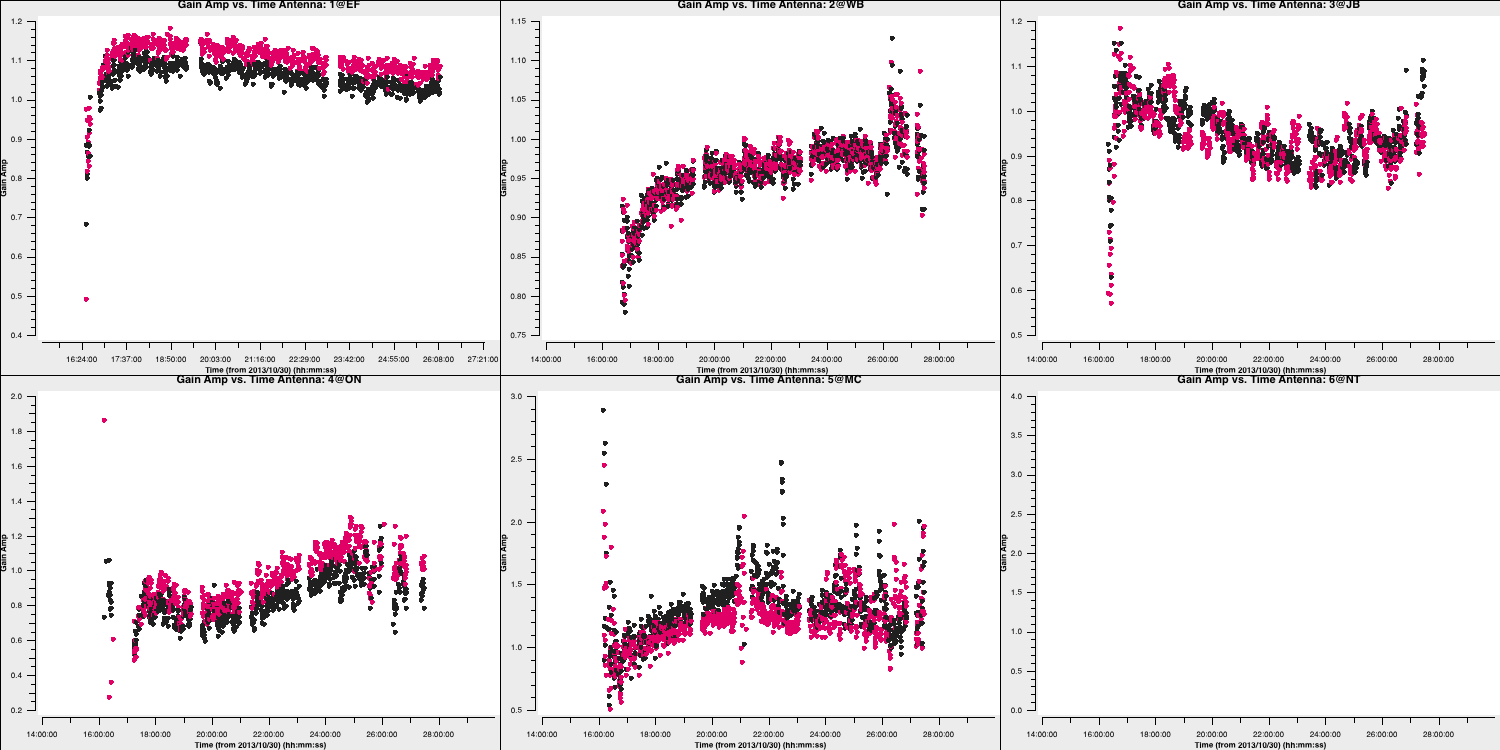As you can see, these solutions are clean and are smoothly varying so must be ok. If you traverse through the solutions, you'll see that the high amplitudes we saw earlier will be corrrected by this table.

Let's apply these to the data:

applycal(vis='NGC660shift.ms',
gaintable='NGC660.ap1',
applymode='calonly')

## 3. Image the calibrated continuum, subtract continuum from the line and make the line cube

### A. Image the calibrated continuum (step 9)

Important: If you performed steps 1-7 you should have NGC660shift.ms, but if not, move or delete any existing NGC660shift.ms and unpack an already made version using tar -zxvf NGC660shift.ms.tgz

We want to image the continuum only, therefore we should use the contchans parameter that we defined earlier so that we avoid the spectral line. In addition, we derived the cellsize in step 3 so this should also be set. In this tclean iteration, we are however going to change the weighting to be Brigg's weighting with a robust of 0. This gives us a better resolution which allows us to distinguish between the core and jet. Take a look at the imaging lectures to learn more about different weighting schemes.

tclean(vis='NGC660shift.ms',
imagename='NGC660_contap1.clean',
field='NGC660',
spw=contchans,
deconvolver='clark',
imsize=[320,320],
weighting='briggs',
robust=0,
cell=cellsize,
niter=200,
interactive=True,
cycleniter=50)

If we use the same boxes as before, we can see that we now have $S_\mathrm{p} \approx 0.1283\,\mathrm{Jy\,beam^{-1}}$, rms noise, $\sigma \approx 0.6\,\mathrm{mJy\,beam^{-1}}$ and so a $\mathrm{S/N}\approx 229$. This is a great improvement upon what we had before.

Inspect the image in the viewer and double check this yourself to work out your S/N. You should also see the core-jet structure appear with our use of the different weighting scheme. The plot below shows the difference in structure that we obtain with natural and briggs (robust=0) weighting on the same data. Note that the peak brightness is lower due to the increased resolution (and smaller synthesised beam).### B. Subtract the continuum and image the line cube (step 10-11)

The next step is to remove the continuum emission from the spectral line absorption/emission. To do this we use a task called uvcontsub. This takes the model of the Fourier Transformed model in the measurement set and subtracts these from the corrected visibility data. We firstly need to put our most recent model into the data set:

ft(vis='NGC660shift.ms',
model='NGC660_contap1.clean.model',
usescratch=True)

And then we remove this model from the visibilities. We select only the continuum channels and interpolate (linearly hence fitorder=1) over the gap where the spectral line is. The continuum subtraction is calculated per integration.

os.system('rm -rf NGC660shift.ms.contsub*')
uvcontsub(vis='NGC660shift.ms',
fitspw=contchans,
fitorder=1)

This produces a new measurement set called NGC660shift.ms.contsub which should have just noise remaining in the contchans.

Now we want to move onto making our first image cube which allows us to determine which components are moving towards and away from us. To get the correct velocities we have to set the line rest frequency. We did this earlier when we estimated the location of the line using slsearch but let's do it again to ensure you understand. Assuming that you only remember the rough rest frequency of the HI 21-cm line, you can get an accurate value using the following command:

slsearch(freqrange=[1.408376,1.424376],
rrlinclude=False,
logfile='NGC660_HIrest.log',
verbose=True,
append=False)

You should get a bunch of lines in the output file NGC660_HIrest.log. Take a look for atomic hydrogen. The output should look something like the following:

SPECIES       RECOMMENDED        CHEMICAL_NAME   FREQUENCY     QUANTUM_NUMBERS  INTENSITY      SMU2      LOGA        EL        EU  LINELIST
...
t-HCOOH                 * Formic Acid             1.418660 43(7,36)-43(7,37)     -9.89870   4.27423   1179.34717  CDMS
H-atom                  * Atomic Hydrogen         1.420410 2S1/2,F=1-0           -9.06120   0.00026 -14.54125   0.00000   0.06817  JPL
g-CH3CH2OH              * gauche-Ethanol          1.420760 35(7,28)-35(7,29),vt  -9.81460   4.41121 -11.68321 641.53949 641.60767  JPL
...

You should find a value of $1.420410\,\mathrm{GHz}$ for HI.

Select the line channels in between the continuum channels in order to image. The default in tclean, which we have used so far, is to average all channels to make a continuum map. Now, use specmode='cube' to image each channel separately (or as determined by parameter width, if you want to average for more sensitivity). Choose the start channel as the end of the first chunk of continuum, and set nchans to the number of central channels which you did not use for continuum (i.e. the number of channels for the output cube, not the original channel number). By default CASA uses the radio convention for converting the fractional frequency shift to velocity ($v$), that is, $$v = c \times \frac{\nu_\mathrm{rest} - \nu_\mathrm{obs}}{\nu_\mathrm{rest}}.$$ We can now make our image cube:

os.system('rm -rf NGC660_line.clean*')
tclean(vis='NGC660shift.ms.contsub',
imagename='NGC660_line.clean',
field='NGC660',
specmode='cube',
start=450,
width=1,
nchan=100,
outframe='LSRK',
restfreq='1.420410GHz',
imsize=[320,320],
cell=cellsize,
weighting='briggs',
robust=0,
niter=1000,
interactive=True,
cycleniter=25)

We want to set a mask for all the channels at once when cleaning otherwise it will take ages to set individual masks for each channel. If the absorption was more complex then we may consider that. You must click the all channels button before generating the bask to do this. We want to clean until all the channels show noise like structure.

We can use imstat just like before to get some statistics. When used on a cube it will provide statistics for the whole cube, and so the channel with the minimum can be identified.

rms=imstat(imagename='NGC660_line.clean.image',
box=offbox)['rms']
peak=imstat(imagename='NGC660_line.clean.image',
box=onbox)['min']
peakchan=imstat(imagename='NGC660_line.clean.image',
box=onbox)['minpos']

print('Max. absorption %6.4f, in chan %3i, rms %6.4f, S/N %6.0f' % (peak, peakchan, rms, abs(peak/rms)))

Take a look at the cube in the viewer and experiment with the look-up table and so on. You can also open the continuum image on top as contours. You should find the following line structure appear which we shall analyse next: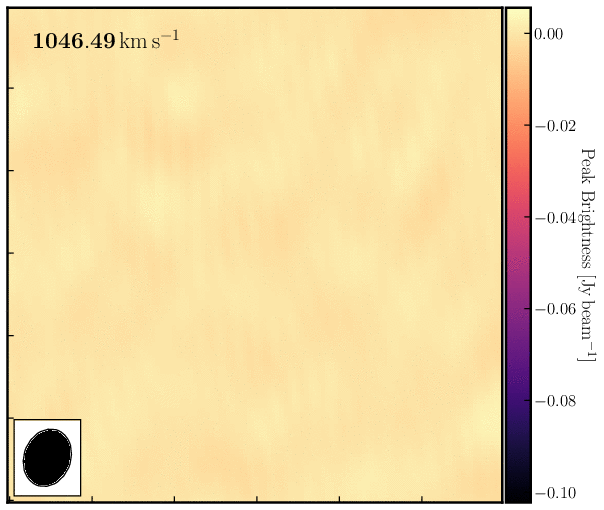## 4. Image cube analysis

By the end of step 11 you should have your own line and continuum images, but if not, untar NGC660_line.clean.image.tgz and NGC660_contap1.clean.image.tgz as described before step 8. Some of the analysis steps here - PV slice, making moments and extracting spectra - can be done interactively in the viewer, which is useful for deciding what parts of the image to include, but it is then better to script using the equivalent tasks so you can repeat the steps.

### A. Make a position-velocity plot (step 12)

You can collapse the spatial dimension along an arbitrary direction and plot the averaged flux density against velocity, known for short as a PV plot. Display NGC660_line.clean.image in the viewer and select channel 60, to decide where to draw the slice, along the jet axis. In the Regions PV tab, change the coordinates to pixels and set the width to 11 pixels. Note the values (to use in the equivalent task) and create the PV plot.We can do the same in a scriptable way using the impv task. In this task we need to define the start and the end of the pv line which we can get from the viewer.

impv(imagename='NGC660_line.clean.image',
outfile='NGC660.pv',
start=[182,142],
end=[133,182],
width=11)

What does this tell us? Well the recessional velocity in the LSRK frame of the host galaxy is around $845\pm1\,\mathrm{km\,s^{-1}}$ therefore, this plot shows that we have a strong jet of HI moving away from the black hole and a weaker jet moving towards us. We can quantify this further by taking moment maps, as we will do in the next section.

### B. Make moments (step 13)

Moment maps are useful as we can use them to derive parameters such as integrated line intensity, centroid velocity and line widths as function of position. The zeroth moment or moment 0 gives us the total intensity of the line ($I_\mathrm{tot}$) and is defined as: $$I_\mathrm{tot}(\alpha,\delta) = \Delta v\sum^{N_\mathrm{chan}}_{i=1}S_\nu(\alpha,\delta,v_i)$$ where $S_\nu(\alpha,\delta,v_i)$ is the flux density per channel. The first moment or moment 1 gives us the intensity-weighted velocity or the mean velocity ($\bar{v}$) and is defined as: $$\overline{v}(\alpha, \delta)=\frac{\sum_{i=1}^{N_{\text { chan }}} v_{i} S_{\nu}\left(\alpha, \delta, \nu_{i}\right)}{\sum_{i=1}^{N_{\text { chan }}} S_{\nu}\left(\alpha, \delta, \nu_{i}\right)}$$ Moments are sensitive to noise so clipping is required. Sum only over the planes of the data cube that contain emission and, since higher order moments depend on lower ones (so progressively noisier), set a conservative intensity threshold for 1st and 2nd moments.

Load the line image cube in the viewer, go to a channel with strong absorption such as 60 and select the moments tool. This will present the spectral profiler. Draw an ellipse round the core and see the spectrum. Shift-click in the spectral profile tool to select the channels covering the line. Select the zeroth moment (total intensity). Note the start and stop channels (approximately) and click on Collapse.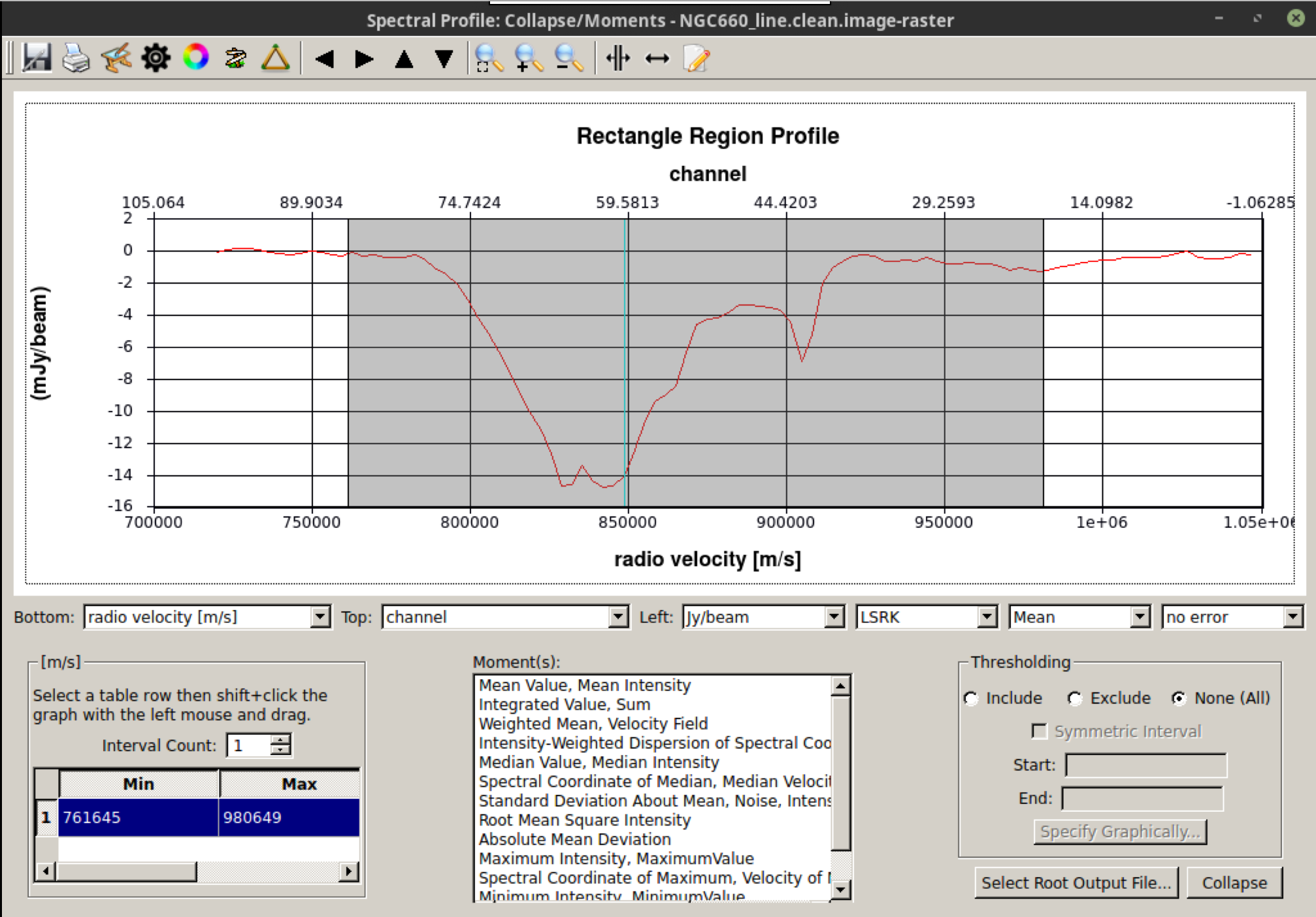We can also script this using the CASA task immoments and we should only use the channels with the line signal

os.system('rm -rf NGC660_line.clean.mom0')
immoments(imagename='NGC660_line.clean.image',
moments=,
chans='33~83',
outfile='NGC660_line.clean.mom0')

When making the first moment, flux-weighted velocity, you need to exclude the noise. About 4 x the rms (see the end of step 9) is about right for the lower limit, as a negative; set a high upper limit to exclude since we are only interested in absorption.

os.system('rm -rf NGC660_line.clean.mom1')
immoments(imagename='NGC660_line.clean.image',
moments=,
chans='33~83',
excludepix=[-0.002,1],
outfile='NGC660_line.clean.mom1')

Open these images in the viewer and adjust the color scales to see what the moment structure looks like.This shows the zeroth moment and the first moment made using a cutoff of $-0.005\,\mathrm{Jy\,beam^{-1}}$, with some tweaking of the color scale in python. You can see a gradient from blue-shifted at the side of the more prominent NE jet, to redshifted towards the weaker counter-jet. This reinforced what we saw when looking through the spectral curve.

### B. Extract and plot spectra (step 14)

One of the steps that we want to do in our analysis is to observe what the HI absorption spectra looks like at different locations across the line of sight. This can give us information on the nature of the gas, such as it's dynamics.

Load the spectral cube in the viewer, go to a channel with strong absorption e.g. 60, and overplot the continuum contours. Click on the spectral profile tool. Draw 3 ellipses on the core and in the direction of the NE jet and the fainter counter-jet. In practice, you might have other information to help decide where it is interesting to extract a spectrum. When you click in one of the ellipses, the spectrum appears in the spectral profile tool. You can also get the coordinates (in pixels) from the Region tab.Scripting uses a tool, ia (for image analysis) to extract the spectra and then we can use raw python to make a plot. This would be particularly convenient to put into a script for future use.

Define the centres of the three positions; I have set all the radii to 6 pixels (what is that in arcsec?) but they don't have to be the same. This is a python dictionary, where the keys ('jet' etc.) are associated with a value (the definition of each circle).

rgns={'jet':'circle[[156pix, 165pix], 6pix]',
'core':'circle[[161pix, 160pix], 6pix]',
'cjet':'circle[[167pix, 154pix], 6pix]',
'rms':'box[[10pix,10pix],[100pix,50pix]]'}

Use the ia tool to get the spectral profile at each of the 3 positions and an off-source noise box and write them to text files.

ia.open('NGC660_line.clean.image')
for r in rgns:
outfile=open(r+'.spec', 'w') # create empty text files called 'jet.spec' etc. in writeable mode
f=ia.getprofile(axis = 3,  # extract the profile and store it in variable f
function = 'flux',
region = rgns[r],
unit='km/s',
for z in zip(f['coords'],f['values']): # python to extract the velocity and flux arrays from f in a suitable format
print >> outfile, z, z # print these to the outfile
outfile.close()

ia.close()

We could have passed the profiles directly to a plotter, but like this, you now have the *.spec files on disk to use as you like. Let's use python to plot the spectra.

import matplotlib.pyplot as plt
cols={'jet':'b','core':'g','cjet':'r','rms':'k'} # The colors for each spectrum (use help(plt.colors) for others)
fig = plt.figure(1,figsize=(9,6))
for i in range(len(rgns)):
v=[];f=[]
for l in open(list(rgns.keys())[i]+'.spec'):  # Read the disk files back into arrays
v.append(float(l.split())) # Array of velocities for x-axis
f.append(float(l.split())) # Array of flux density values for y-axis
ax.plot(v,f,color=cols[list(rgns.keys())[i]],label=r'%s'%list(rgns.keys())[i])  # Plot the spectra
ax.set_xlabel('Velocity (LSR, km/s)')
ax.set_ylabel('Flux density per channel per 12-mas radius')
ax.set_title('NGC660 HI velocity profiles')
ax.legend() # add the legend to show what each line stands for
fig.savefig('NGC660_HIvelocityprofiles.pdf',bbox_inches='tight') # Write the plot to a pdf
plt.show()### C. Make an optical depth map (step 15)

The optical depth tells us how much energy has been lost by travelling through a dense medium. This allows us to probe where the obscuration is greatest across the source that is illuminating this dense medium. In our case, we have our bright continuum superimposed upon the absoprtion of light by the HI transition. We can use these to work out the optical depth.

In the optically thin approximation, the optical depth ($\tau$) is given by, $$\tau = -\ln\left(\frac{|I_L|}{I_C}\right),$$ where $I_L$ is the flux density of the continuum-subtracted line and $I_C$ is the flux density of the continuum.

This can be done for single planes of the cube or for an average of many planes. Make an average of the most intense absorption, e.g. below half-minimum:

os.system('rm -rf NGC660_line.mean')
immoments(imagename='NGC660_line.clean.image',
moments=[-1],
chans='55~69',    # main peak FWHM
excludepix=[-0.005,1],
outfile='NGC660_line.mean')

Expressions such as for optical depth can be coded in immath:

os.system('rm -rf NGC660.tau')
immath(imagename=['NGC660_contap1.clean.image','NGC660_line.mean'],
mode='evalexpr',
expr='-1.*log(-1.*IM1/IM0)',
outfile='NGC660.tau')

In the viewer, set the range of values to [0,1] to exclude noise pixels and you should seeHooray, this concludes the spectral line workshop. You have performed self-calibration and imaging of a spectral cube and should have learnt about how to remove the continuum emission. On top of this, we have performed some analysis on the spectral cube via moments, pv maps and optical depth calculations. This analysis has revealed that the NGC660 outburst has two components which are moving towards and away from the central black hole. Based upon the optical depth maps, the obscuring fraction of HI is a little higher towards the centre of the object indicating that the gas is denser and could be fuelling this outburst.